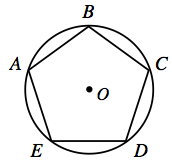### Home > INT2 > Chapter 10 > Lesson 10.2.2 > Problem10-67

10-67.

$ABCDE$ is a regular pentagon inscribed in $⊙O$, meaning that each of its five vertices lie on the circle.

1. Draw a diagram of $ABCDE$ and $⊙O$ on your paper.2. What is $m∠EDC$? How did you determine your answer?

Recall that the sum of the interior angles of a regular pentagon is $540°$.

3. What is $m∠BOC$? What relationship did you use?

Notice that $m∠BOC$ is one-fifth of the circle.

4. What is m$\overarc { E B C }$? Show more than one way to calculate this measure.

What is the relationship between $m∠BOC$ and the arc in question?

$216°$